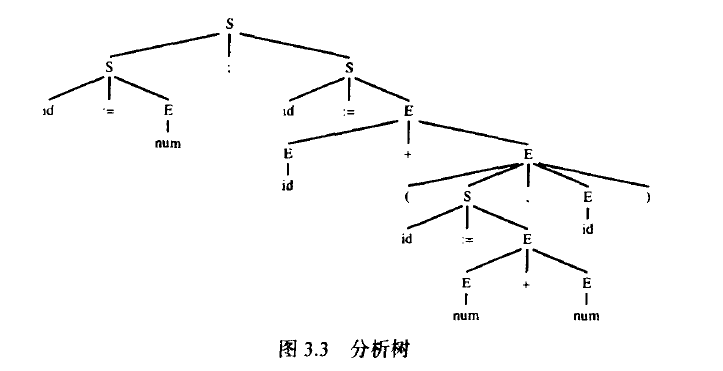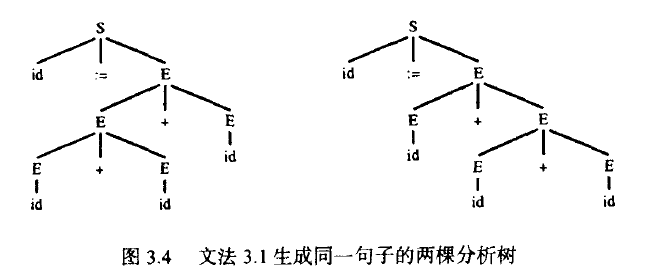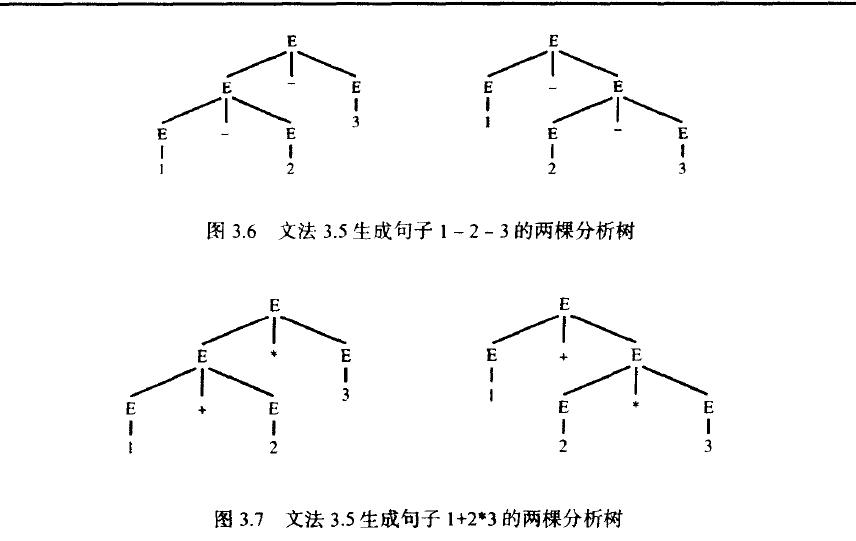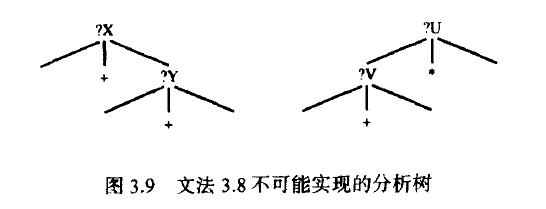# 语法分析

digits=[0-9] +
sum=(digits“+") *digits


digits=[0-9] +
Sum=expr“+"expr
expr="("sum") " | digits


(109+23)
61
(1+(250+3))


expr="("expr"+"expr") " | digits


expr="("("("expr"+"expr") " | digits) "+"expr") " | digits


expr=ab(c|d)e


aux=c d
expr=a b aux e


aux=c
aux=d
expr=a b aux e


expr=(abc) *


expr=(abc) expr
expr=epolison


# 上下文无关文法

symbol→symbol symbol...symbol


id print num+：=；


id：=num； id：=id+(id：=num+num， id)


## 文法 3.1 直线程序的语法

S-> S:S
S-> id := E
S-> print(L)

E-> id
E-> num
E-> E+E
E-> (S, E)
L-> E
L-> L, E


a：=7；
b：=+(d：=5+6，d)


(终结符的) 记号类型为id， num，=，等等，名字(a，b，c，d)和数字(7，5，6)对应着一些有语义价值的记号

## 推导

• 推导3.2
S'
S；S'
S'；id：=E
id：=E'；id：=E
id：=num； id：=E'
id：=num； id：=E+E'
id：=num； id：=E'+(S， E)
id：=num：id：=id+(S'，E)
id：=num； id：=id+(id：=E'， E)
id：=num； id：=id+(id：=E+E， E')
id：=num； id：=id+(id：=E'+E， id)
id：=num； id：=id+(id：=num+E'， id)
id：=num； id：=id+(id：=num+num， id)


S'
S': S
id：=E'：S
id：=num； S'
id：=num； id：=E'
id：=num； id：=E'+E


## 分析树## 二义性文法• 文法3.5
E→id
E→num
E→E*E
E→E/E
E→E+E
E→E-E
E→(E)## 非二义性文法

• 文法3.8
E→E+T
T→T*F
F→id
E→E-T
T→T/F
F→num
E→T
T→F
F→(E)


 该文法接收与二义性文法同样的字符集，但是现在每个句 子都对应一个确定的分析树。## 文件结束符

E→E+T
E→E-T
E→T

T→T*F
T→T/F
T→F

F→id
F→num
F→(E)


《现代编译原理 java》